# Selina Solutions Concise Maths Class 7 Chapter 8: Percent and Percentage Exercise 8A

Selina Solutions Concise Maths Class 7 Chapter 8 Percent and Percentage Exercise 8A covers major topics like basic concept and the method of expressing a given statement as percent. In a subject like Mathematics, the students should first understand the important concepts, as per ICSE syllabus. The solutions contain important formulas and shortcut tricks, which are used in solving the exercise wise problems. After solving the textbook problems, students can cross check their answers with Selina Solutions Concise Maths Class 7 Chapter 8 Percent and Percentage Exercise 8A PDF, using the links provided below.

## Selina Solutions Concise Maths Class 7 Chapter 8: Percent and Percentage Exercise 8A Download PDF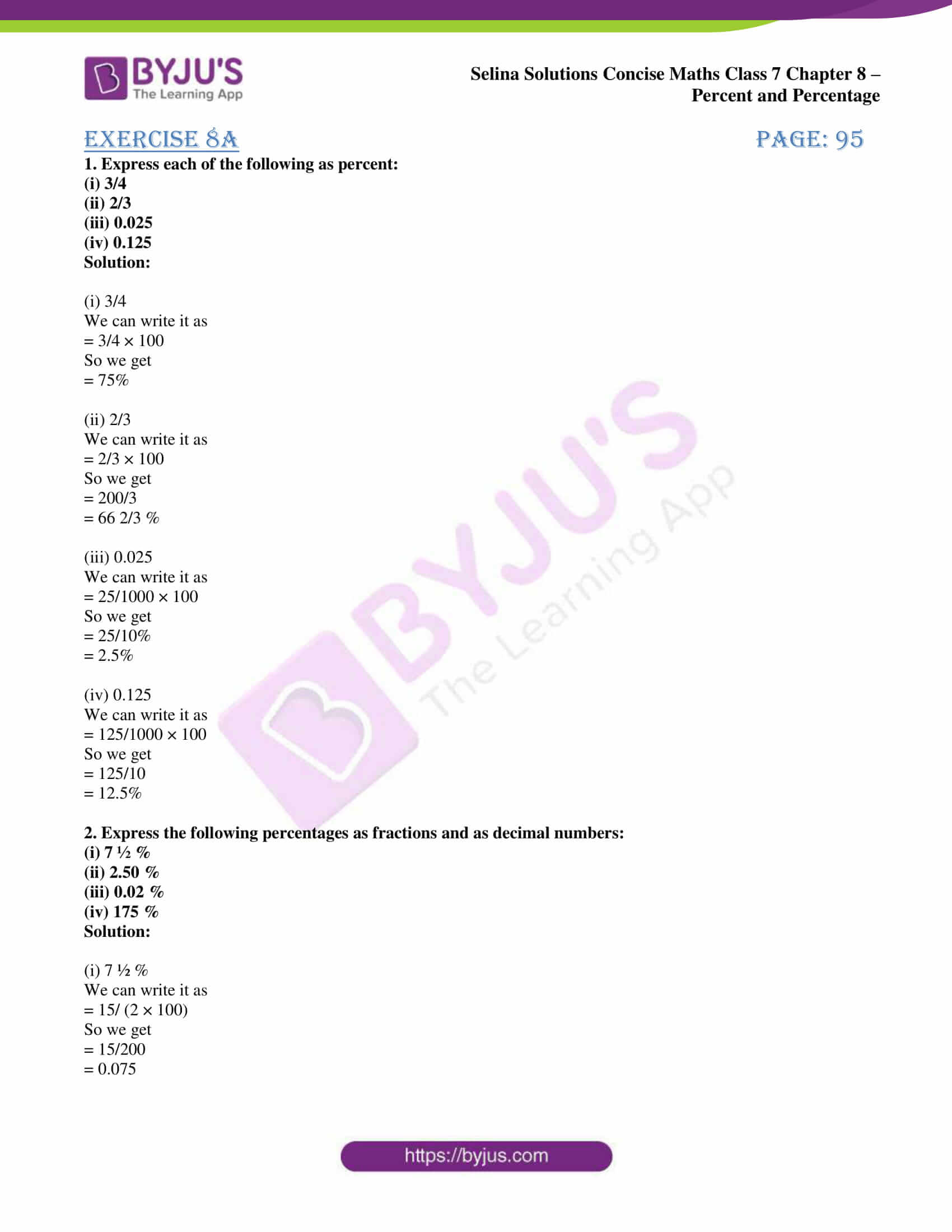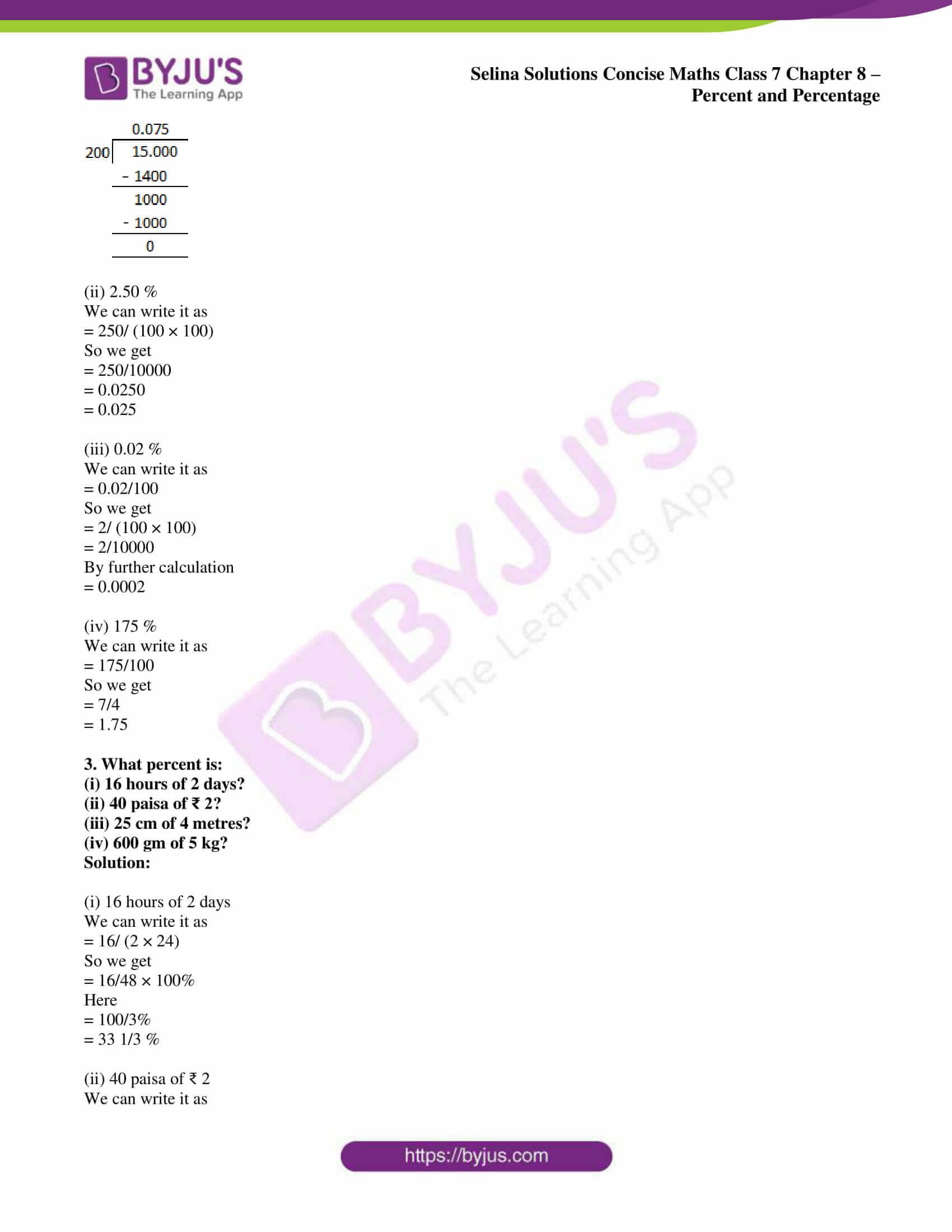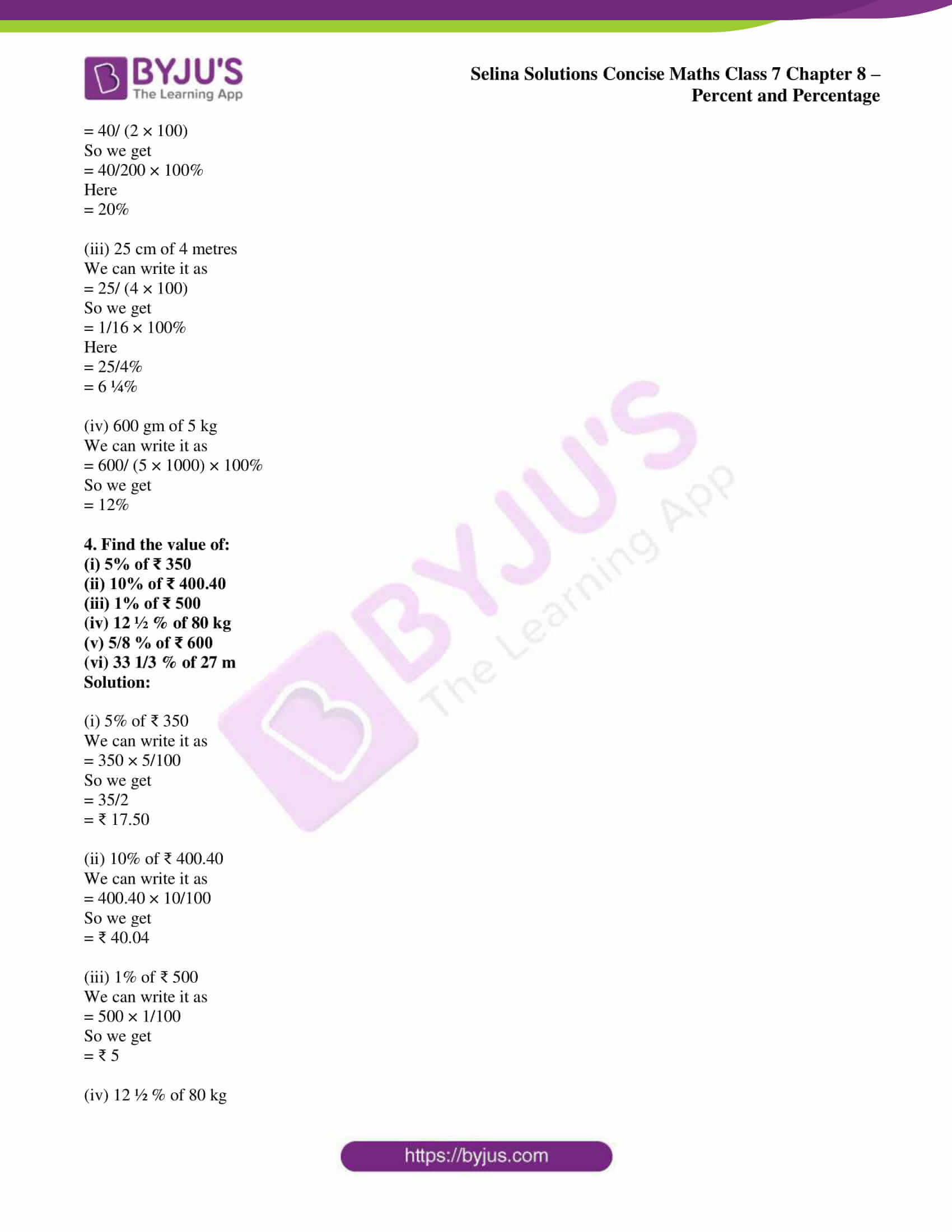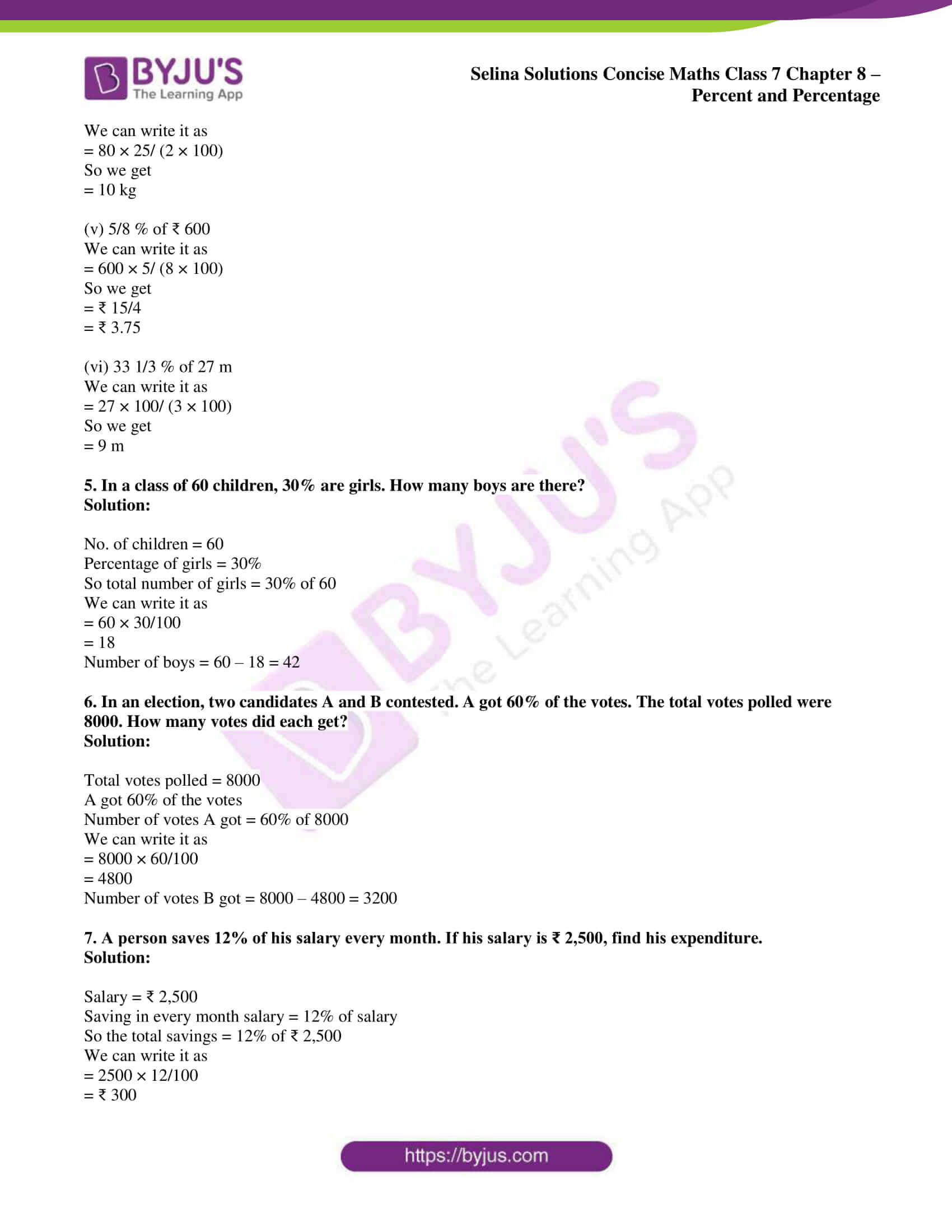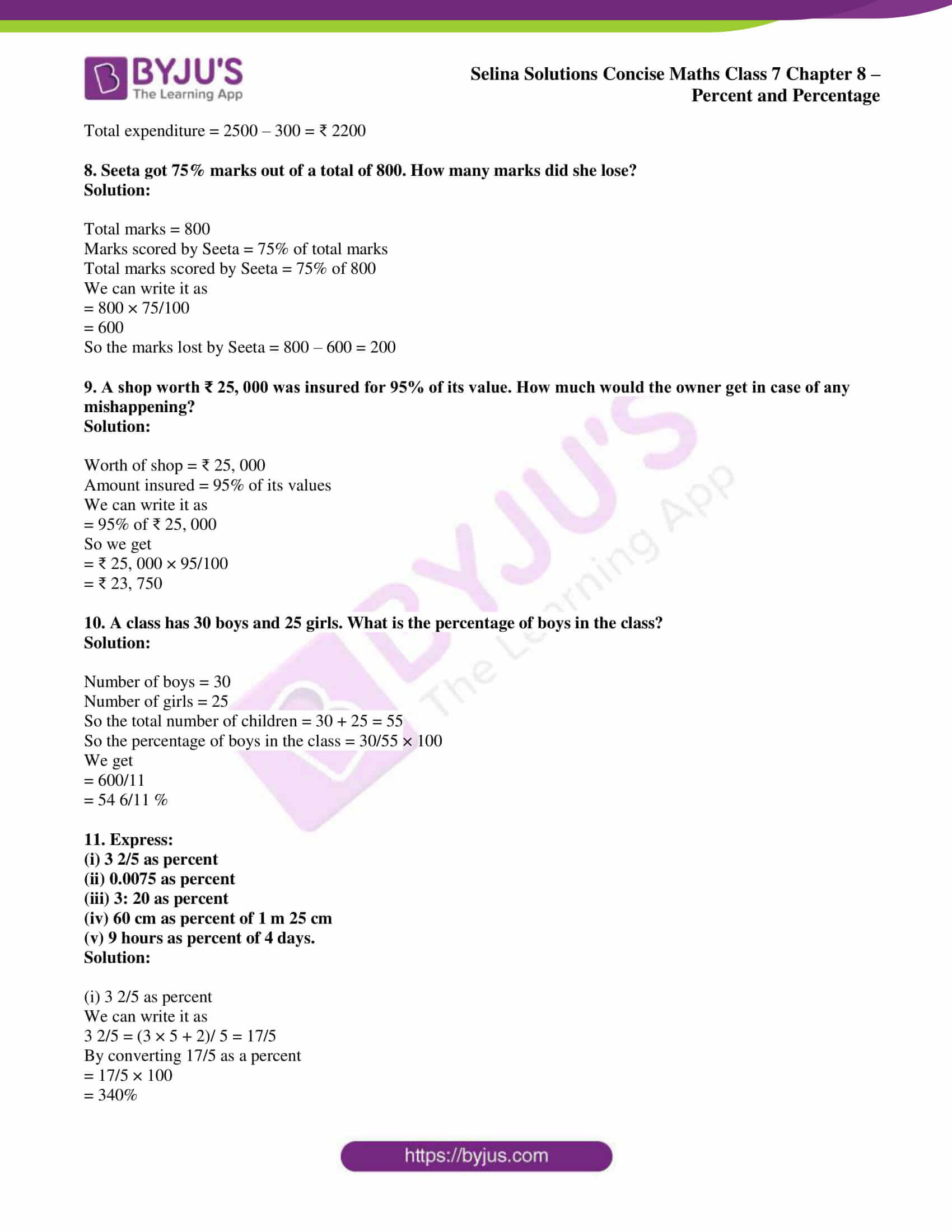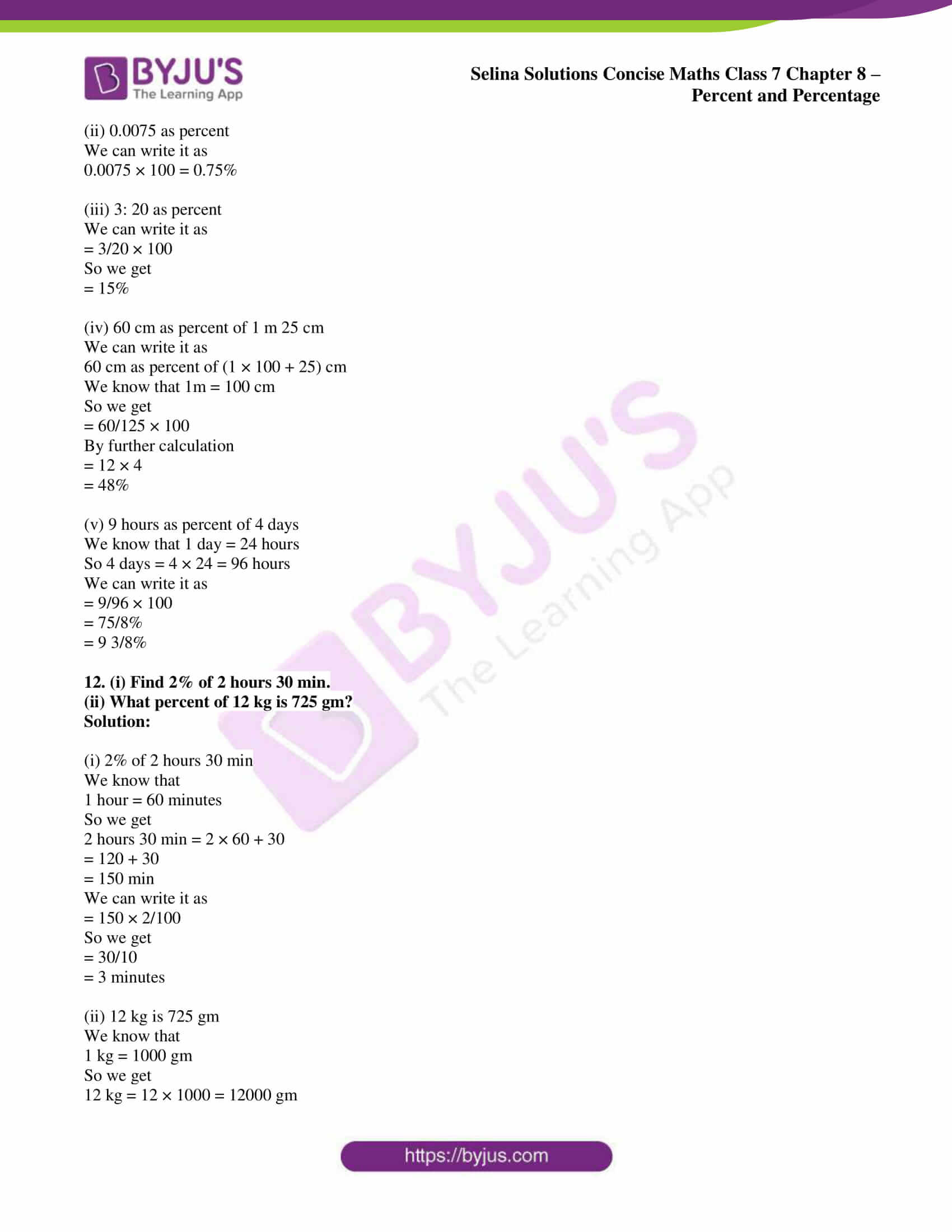### Access Selina Solutions Concise Maths Class 7 Chapter 8: Percent and Percentage Exercise 8A

1. Express each of the following as percent:

(i) 3/4

(ii) 2/3

(iii) 0.025

(iv) 0.125

Solution:

(i) 3/4

We can write it as

= 3/4 × 100

So we get

= 75%

(ii) 2/3

We can write it as

= 2/3 × 100

So we get

= 200/3

= 66 2/3 %

(iii) 0.025

We can write it as

= 25/1000 × 100

So we get

= 25/10%

= 2.5%

(iv) 0.125

We can write it as

= 125/1000 × 100

So we get

= 125/10

= 12.5%

2. Express the following percentages as fractions and as decimal numbers:

(i) 7 ½ %

(ii) 2.50 %

(iii) 0.02 %

(iv) 175 %

Solution:

(i) 7 ½ %

We can write it as

= 15/ (2 × 100)

So we get

= 15/200

= 0.075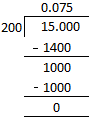(ii) 2.50 %

We can write it as

= 250/ (100 × 100)

So we get

= 250/10000

= 0.0250

= 0.025

(iii) 0.02 %

We can write it as

= 0.02/100

So we get

= 2/ (100 × 100)

= 2/10000

By further calculation

= 0.0002

(iv) 175 %

We can write it as

= 175/100

So we get

= 7/4

= 1.75

3. What percent is:

(i) 16 hours of 2 days?

(ii) 40 paisa of ₹ 2?

(iii) 25 cm of 4 metres?

(iv) 600 gm of 5 kg?

Solution:

(i) 16 hours of 2 days

We can write it as

= 16/ (2 × 24)

So we get

= 16/48 × 100%

Here

= 100/3%

= 33 1/3 %

(ii) 40 paisa of ₹ 2

We can write it as

= 40/ (2 × 100)

So we get

= 40/200 × 100%

Here

= 20%

(iii) 25 cm of 4 metres

We can write it as

= 25/ (4 × 100)

So we get

= 1/16 × 100%

Here

= 25/4%

= 6 ¼%

(iv) 600 gm of 5 kg

We can write it as

= 600/ (5 × 1000) × 100%

So we get

= 12%

4. Find the value of:

(i) 5% of ₹ 350

(ii) 10% of ₹ 400.40

(iii) 1% of ₹ 500

(iv) 12 ½ % of 80 kg

(v) 5/8 % of ₹ 600

(vi) 33 1/3 % of 27 m

Solution:

(i) 5% of ₹ 350

We can write it as

= 350 × 5/100

So we get

= 35/2

= ₹ 17.50

(ii) 10% of ₹ 400.40

We can write it as

= 400.40 × 10/100

So we get

= ₹ 40.04

(iii) 1% of ₹ 500

We can write it as

= 500 × 1/100

So we get

= ₹ 5

(iv) 12 ½ % of 80 kg

We can write it as

= 80 × 25/ (2 × 100)

So we get

= 10 kg

(v) 5/8 % of ₹ 600

We can write it as

= 600 × 5/ (8 × 100)

So we get

= ₹ 15/4

= ₹ 3.75

(vi) 33 1/3 % of 27 m

We can write it as

= 27 × 100/ (3 × 100)

So we get

= 9 m

5. In a class of 60 children, 30% are girls. How many boys are there?

Solution:

No. of children = 60

Percentage of girls = 30%

So total number of girls = 30% of 60

We can write it as

= 60 × 30/100

= 18

Number of boys = 60 – 18 = 42

6. In an election, two candidates A and B contested. A got 60% of the votes. The total votes polled were 8000. How many votes did each get?

Solution:

A got 60% of the votes

Number of votes A got = 60% of 8000

We can write it as

= 8000 × 60/100

= 4800

Number of votes B got = 8000 – 4800 = 3200

7. A person saves 12% of his salary every month. If his salary is ₹ 2,500, find his expenditure.

Solution:

Salary = ₹ 2,500

Saving in every month salary = 12% of salary

So the total savings = 12% of ₹ 2,500

We can write it as

= 2500 × 12/100

= ₹ 300

Total expenditure = 2500 – 300 = ₹ 2200

8. Seeta got 75% marks out of a total of 800. How many marks did she lose?

Solution:

Total marks = 800

Marks scored by Seeta = 75% of total marks

Total marks scored by Seeta = 75% of 800

We can write it as

= 800 × 75/100

= 600

So the marks lost by Seeta = 800 – 600 = 200

9. A shop worth ₹ 25, 000 was insured for 95% of its value. How much would the owner get in case of any mishappening?

Solution:

Worth of shop = ₹ 25, 000

Amount insured = 95% of its values

We can write it as

= 95% of ₹ 25, 000

So we get

= ₹ 25, 000 × 95/100

= ₹ 23, 750

10. A class has 30 boys and 25 girls. What is the percentage of boys in the class?

Solution:

Number of boys = 30

Number of girls = 25

So the total number of children = 30 + 25 = 55

So the percentage of boys in the class = 30/55 × 100

We get

= 600/11

= 54 6/11 %

11. Express:

(i) 3 2/5 as percent

(ii) 0.0075 as percent

(iii) 3: 20 as percent

(iv) 60 cm as percent of 1 m 25 cm

(v) 9 hours as percent of 4 days.

Solution:

(i) 3 2/5 as percent

We can write it as

3 2/5 = (3 × 5 + 2)/ 5 = 17/5

By converting 17/5 as a percent

= 17/5 × 100

= 340%

(ii) 0.0075 as percent

We can write it as

0.0075 × 100 = 0.75%

(iii) 3: 20 as percent

We can write it as

= 3/20 × 100

So we get

= 15%

(iv) 60 cm as percent of 1 m 25 cm

We can write it as

60 cm as percent of (1 × 100 + 25) cm

We know that 1m = 100 cm

So we get

= 60/125 × 100

By further calculation

= 12 × 4

= 48%

(v) 9 hours as percent of 4 days

We know that 1 day = 24 hours

So 4 days = 4 × 24 = 96 hours

We can write it as

= 9/96 × 100

= 75/8%

= 9 3/8%

12. (i) Find 2% of 2 hours 30 min.

(ii) What percent of 12 kg is 725 gm?

Solution:

(i) 2% of 2 hours 30 min

We know that

1 hour = 60 minutes

So we get

2 hours 30 min = 2 × 60 + 30

= 120 + 30

= 150 min

We can write it as

= 150 × 2/100

So we get

= 30/10

= 3 minutes

(ii) 12 kg is 725 gm

We know that

1 kg = 1000 gm

So we get

12 kg = 12 × 1000 = 12000 gm

We can write it as

= 725/12000 × 100

So we get

= 725/120

= 145/24%

= 6 1/24%